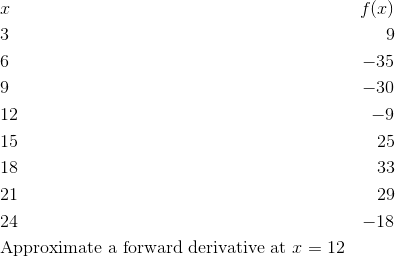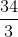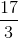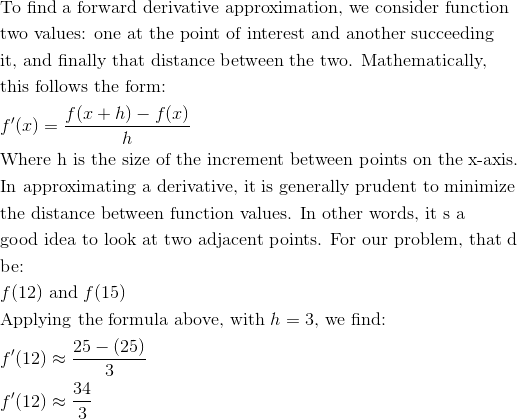# AP Calculus AB : Approximate rate of change from graphs and tables of values

## Example Questions

← Previous 1 3 4 5

### Example Question #1 : Approximate Rate Of Change From Graphs And Tables Of Values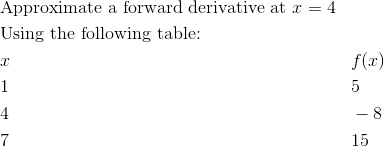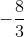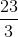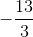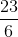Explanation: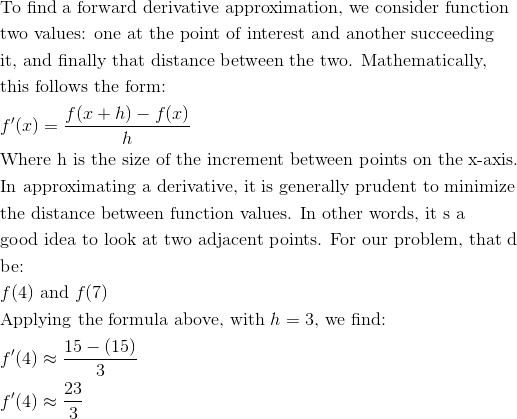### Example Question #2 : Approximate Rate Of Change From Graphs And Tables Of Values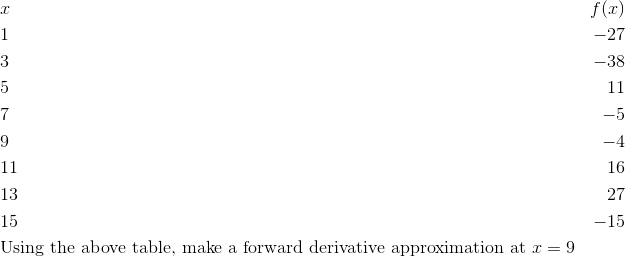Explanation: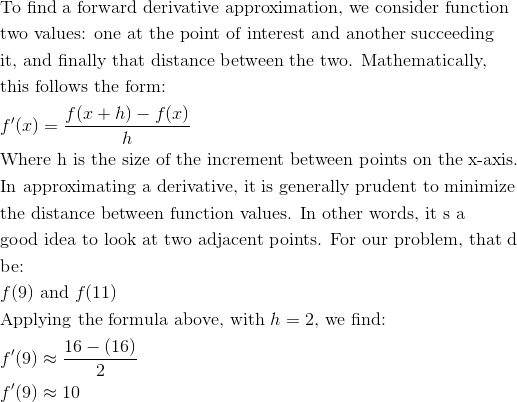### Example Question #3 : Approximate Rate Of Change From Graphs And Tables Of Values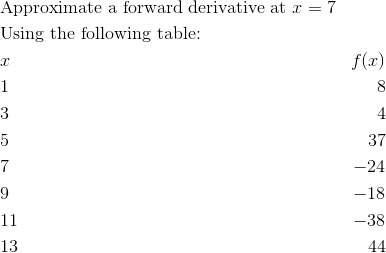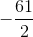Explanation: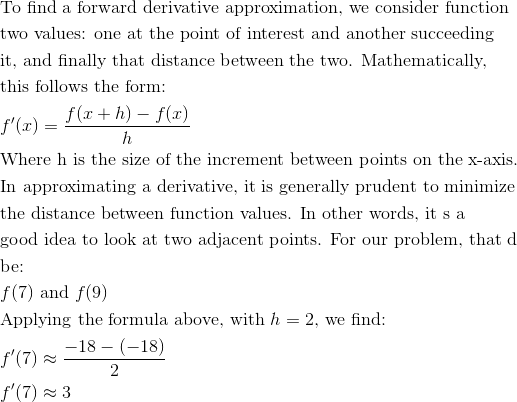### Example Question #4 : Approximate Rate Of Change From Graphs And Tables Of Values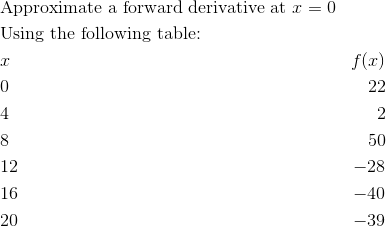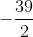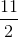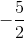Explanation: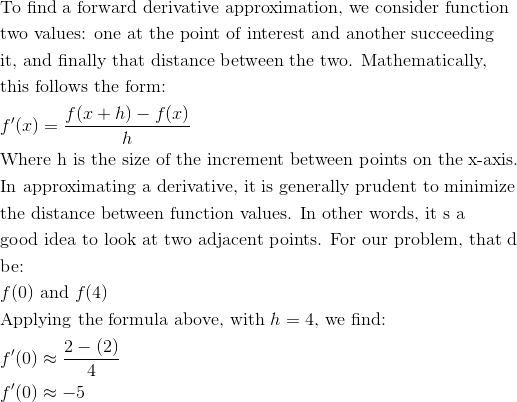### Example Question #5 : Approximate Rate Of Change From Graphs And Tables Of Values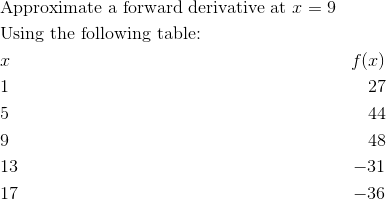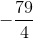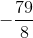Explanation: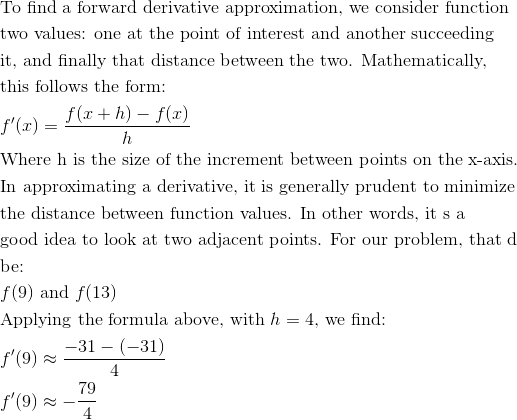### Example Question #6 : Approximate Rate Of Change From Graphs And Tables Of Values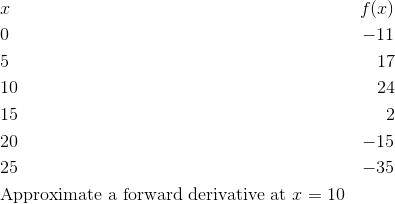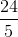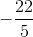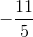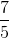Explanation: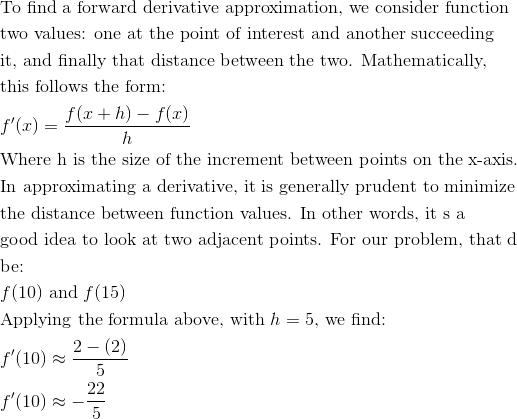### Example Question #7 : Approximate Rate Of Change From Graphs And Tables Of Values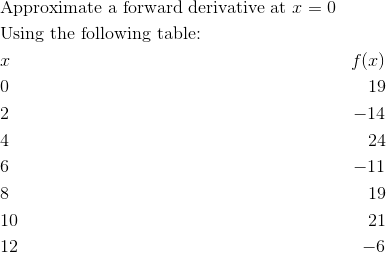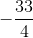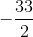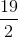Explanation: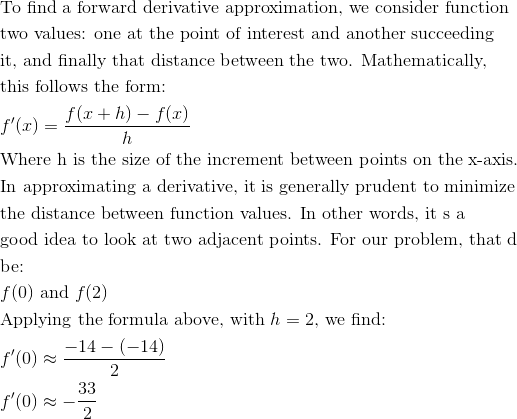### Example Question #8 : Approximate Rate Of Change From Graphs And Tables Of Values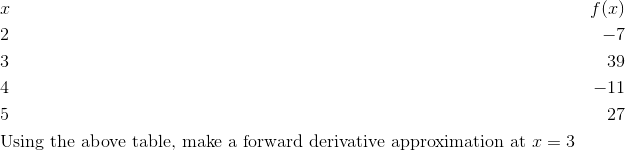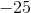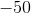Explanation: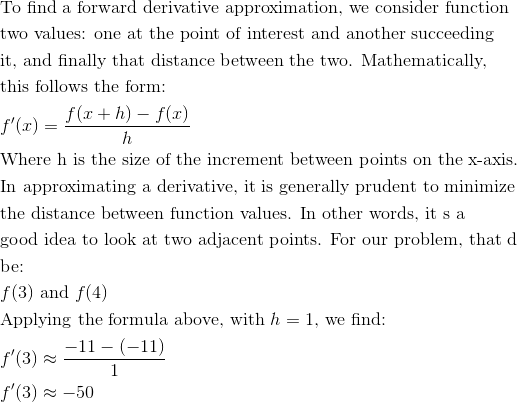### Example Question #9 : Approximate Rate Of Change From Graphs And Tables Of Values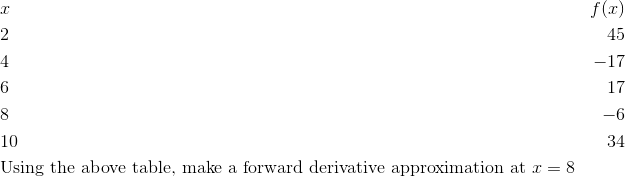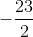Explanation: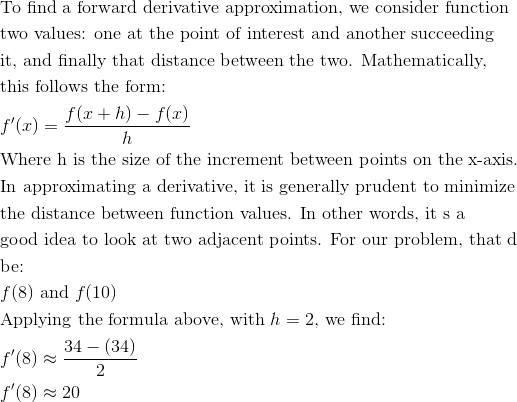### Example Question #1 : Approximate Rate Of Change From Graphs And Tables Of Values